# 1.6 Add and subtract fractions  (Page 3/4)

 Page 3 / 4

Simplify: $\frac{3a}{4}-\phantom{\rule{0.2em}{0ex}}\frac{8}{9}$ $\frac{3a}{4}·\frac{8}{9}.$

$\frac{27a-32}{36}$ $\frac{2a}{3}$

Simplify: $\frac{4k}{5}-\phantom{\rule{0.2em}{0ex}}\frac{1}{6}$ $\frac{4k}{5}·\frac{1}{6}.$

$\frac{24k-5}{30}$ $\frac{2k}{15}$

## Use the order of operations to simplify complex fractions

We have seen that a complex fraction is a fraction in which the numerator or denominator contains a fraction. The fraction bar indicates division . We simplified the complex fraction $\frac{\frac{3}{4}}{\frac{5}{8}}$ by dividing $\frac{3}{4}$ by $\frac{5}{8}.$

Now we’ll look at complex fractions where the numerator or denominator contains an expression that can be simplified. So we first must completely simplify the numerator and denominator separately using the order of operations. Then we divide the numerator by the denominator.

## How to simplify complex fractions

Simplify: $\frac{{\left(\frac{1}{2}\right)}^{2}}{4+{3}^{2}}.$

## Solution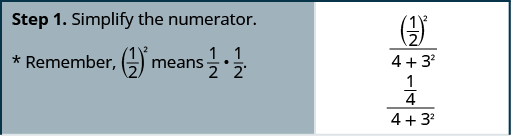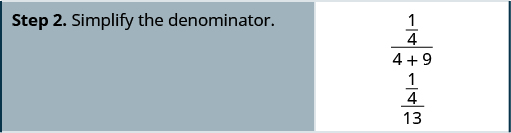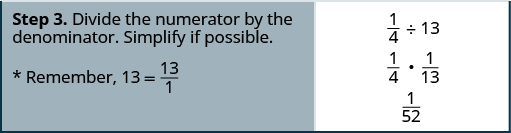Simplify: $\frac{{\left(\frac{1}{3}\right)}^{2}}{{2}^{3}+2}.$

$\frac{1}{90}$

Simplify: $\frac{1+{4}^{2}}{{\left(\frac{1}{4}\right)}^{2}}.$

$272$

## Simplify complex fractions.

1. Simplify the numerator.
2. Simplify the denominator.
3. Divide the numerator by the denominator. Simplify if possible.

Simplify: $\frac{\frac{1}{2}+\frac{2}{3}}{\frac{3}{4}-\phantom{\rule{0.2em}{0ex}}\frac{1}{6}}.$

## Solution

It may help to put parentheses around the numerator and the denominator.

$\begin{array}{cccccc}& & & & & \frac{\left(\frac{1}{2}+\frac{2}{3}\right)}{\left(\frac{3}{4}-\phantom{\rule{0.2em}{0ex}}\frac{1}{6}\right)}\hfill \\ \\ \\ \begin{array}{c}\text{Simplify the numerator (LCD = 6)}\hfill \\ \text{and simplify the denominator (LCD = 12).}\hfill \end{array}\hfill & & & & & \hfill \frac{\left(\frac{3}{6}+\frac{4}{6}\right)}{\left(\frac{9}{12}-\phantom{\rule{0.2em}{0ex}}\frac{2}{12}\right)}\hfill \\ \\ \\ \text{Simplify.}\hfill & & & & & \hfill \frac{\left(\frac{7}{6}\right)}{\left(\frac{7}{12}\right)}\hfill \\ \\ \\ \text{Divide the numerator by the denominator.}\hfill & & & & & \hfill \frac{7}{6}÷\frac{7}{12}\hfill \\ \\ \\ \text{Simplify.}\hfill & & & & & \hfill \frac{7}{6}·\frac{12}{7}\hfill \\ \\ \\ \text{Divide out common factors.}\hfill & & & & & \hfill \frac{7·6·2}{6·7}\hfill \\ \\ \\ \text{Simplify.}\hfill & & & & & \hfill 2\hfill \end{array}$

Simplify: $\frac{\frac{1}{3}+\frac{1}{2}}{\frac{3}{4}-\phantom{\rule{0.2em}{0ex}}\frac{1}{3}}.$

2

Simplify: $\frac{\frac{2}{3}-\phantom{\rule{0.2em}{0ex}}\frac{1}{2}}{\frac{1}{4}+\frac{1}{3}}.$

$\frac{2}{7}$

## Evaluate variable expressions with fractions

We have evaluated expressions before, but now we can evaluate expressions with fractions. Remember, to evaluate an expression, we substitute the value of the variable into the expression and then simplify.

Evaluate $x+\frac{1}{3}$ when $x=-\phantom{\rule{0.2em}{0ex}}\frac{1}{3}$ $x=-\phantom{\rule{0.2em}{0ex}}\frac{3}{4}.$

1. To evaluate $x+\frac{1}{3}$ when $x=-\phantom{\rule{0.2em}{0ex}}\frac{1}{3},$ substitute $-\phantom{\rule{0.2em}{0ex}}\frac{1}{3}$ for $x$ in the expression.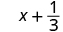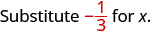Simplify. $\phantom{\rule{18em}{0ex}}$ 0

2. To evaluate $x+\frac{1}{3}$ when $x=-\phantom{\rule{0.2em}{0ex}}\frac{3}{4},$ we substitute $-\phantom{\rule{0.2em}{0ex}}\frac{3}{4}$ for x in the expression.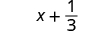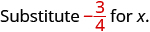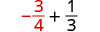Rewrite as equivalent fractions with the LCD, 12.Simplify.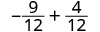Add. $-\phantom{\rule{0.2em}{0ex}}\frac{5}{12}$

Evaluate $x+\frac{3}{4}$ when $x=-\phantom{\rule{0.2em}{0ex}}\frac{7}{4}$ $x=-\phantom{\rule{0.2em}{0ex}}\frac{5}{4}.$

$-1$ $-\phantom{\rule{0.2em}{0ex}}\frac{1}{2}$

Evaluate $y+\frac{1}{2}$ when $y=\frac{2}{3}$ $y=-\phantom{\rule{0.2em}{0ex}}\frac{3}{4}.$

$\frac{7}{6}$ $-\phantom{\rule{0.2em}{0ex}}\frac{1}{12}$

Evaluate $-\phantom{\rule{0.2em}{0ex}}\frac{5}{6}-y$ when $y=-\phantom{\rule{0.2em}{0ex}}\frac{2}{3}.$

## Solution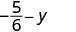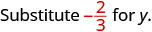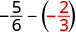Rewrite as equivalent fractions with the LCD, 6.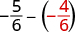Subtract.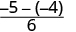Simplify. $-\phantom{\rule{0.2em}{0ex}}\frac{1}{6}$

Evaluate $-\phantom{\rule{0.2em}{0ex}}\frac{1}{2}-y$ when $y=-\phantom{\rule{0.2em}{0ex}}\frac{1}{4}.$

$-\phantom{\rule{0.2em}{0ex}}\frac{1}{4}$

Evaluate $-\phantom{\rule{0.2em}{0ex}}\frac{3}{8}-y$ when $x=-\phantom{\rule{0.2em}{0ex}}\frac{5}{2}.$

$-\phantom{\rule{0.2em}{0ex}}\frac{17}{8}$

Evaluate $2{x}^{2}y$ when $x=\frac{1}{4}$ and $y=-\phantom{\rule{0.2em}{0ex}}\frac{2}{3}.$

## Solution

Substitute the values into the expression.

 $2{x}^{2}y$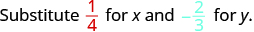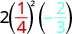Simplify exponents first. $2\left(\frac{1}{16}\right)\left(-\phantom{\rule{0.2em}{0ex}}\frac{2}{3}\right)$ Multiply. Divide out the common factors. Notice we write 16 as $2\cdot 2\cdot 4$ to make it easy to remove common factors. $-\phantom{\rule{0.2em}{0ex}}\frac{\overline{)2}\cdot 1\cdot \overline{)2}}{\overline{)2}\cdot \overline{)2}\cdot 4\cdot 3}$ Simplify. $-\phantom{\rule{0.2em}{0ex}}\frac{1}{12}$

Evaluate $3a{b}^{2}$ when $a=-\phantom{\rule{0.2em}{0ex}}\frac{2}{3}$ and $b=-\phantom{\rule{0.2em}{0ex}}\frac{1}{2}.$

$-\phantom{\rule{0.2em}{0ex}}\frac{1}{2}$

Evaluate $4{c}^{3}d$ when $c=-\phantom{\rule{0.2em}{0ex}}\frac{1}{2}$ and $d=-\phantom{\rule{0.2em}{0ex}}\frac{4}{3}.$

$\frac{2}{3}$

The next example will have only variables, no constants.

Evaluate $\frac{p+q}{r}$ when $p=-4,q=-2,\phantom{\rule{0.2em}{0ex}}\text{and}\phantom{\rule{0.2em}{0ex}}r=8.$

## Solution

To evaluate $\frac{p+q}{r}$ when $p=-4,q=-2,\phantom{\rule{0.2em}{0ex}}\text{and}\phantom{\rule{0.2em}{0ex}}r=8,$ we substitute the values into the expression.

 $\frac{p+q}{r}$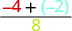Add in the numerator first. $\frac{-6}{8}$ Simplify. $-\phantom{\rule{0.2em}{0ex}}\frac{3}{4}$

Evaluate $\frac{a+b}{c}$ when $a=-8,b=-7,\phantom{\rule{0.2em}{0ex}}\text{and}\phantom{\rule{0.2em}{0ex}}c=6.$

$-\phantom{\rule{0.2em}{0ex}}\frac{5}{2}$

Evaluate $\frac{x+y}{z}$ when $x=9,y=-18,\phantom{\rule{0.2em}{0ex}}\text{and}\phantom{\rule{0.2em}{0ex}}z=-6.$

$\frac{3}{2}$

## Key concepts

• Fraction Addition and Subtraction: If $a,b,\phantom{\rule{0.2em}{0ex}}\text{and}\phantom{\rule{0.2em}{0ex}}c$ are numbers where $c\ne 0,$ then
$\frac{a}{c}+\frac{b}{c}=\frac{a+b}{c}$ and $\frac{a}{c}-\phantom{\rule{0.2em}{0ex}}\frac{b}{c}=\frac{a-b}{c}.$
To add or subtract fractions, add or subtract the numerators and place the result over the common denominator.
• Strategy for Adding or Subtracting Fractions
1. Do they have a common denominator?
Yes—go to step 2.
No—Rewrite each fraction with the LCD (Least Common Denominator). Find the LCD. Change each fraction into an equivalent fraction with the LCD as its denominator.
2. Add or subtract the fractions.
3. Simplify, if possible. To multiply or divide fractions, an LCD IS NOT needed. To add or subtract fractions, an LCD IS needed.
• Simplify Complex Fractions
1. Simplify the numerator.
2. Simplify the denominator.
3. Divide the numerator by the denominator. Simplify if possible.

## Practice makes perfect

Add and Subtract Fractions with a Common Denominator

$\frac{6}{13}+\frac{5}{13}$

$\frac{11}{13}$

$\frac{4}{15}+\frac{7}{15}$

$\frac{x}{4}+\frac{3}{4}$

$\frac{x+3}{4}$

$\frac{8}{q}+\frac{6}{q}$

$-\phantom{\rule{0.2em}{0ex}}\frac{3}{16}+\left(-\phantom{\rule{0.2em}{0ex}}\frac{7}{16}\right)$

$-\phantom{\rule{0.2em}{0ex}}\frac{5}{8}$

$-\phantom{\rule{0.2em}{0ex}}\frac{5}{16}+\left(-\phantom{\rule{0.2em}{0ex}}\frac{9}{16}\right)$

$-\phantom{\rule{0.2em}{0ex}}\frac{8}{17}+\frac{15}{17}$

$\frac{7}{17}$

$-\phantom{\rule{0.2em}{0ex}}\frac{9}{19}+\frac{17}{19}$

$\frac{6}{13}+\left(-\phantom{\rule{0.2em}{0ex}}\frac{10}{13}\right)+\left(-\phantom{\rule{0.2em}{0ex}}\frac{12}{13}\right)$

$-\phantom{\rule{0.2em}{0ex}}\frac{16}{13}$

$\frac{5}{12}+\left(-\phantom{\rule{0.2em}{0ex}}\frac{7}{12}\right)+\left(-\phantom{\rule{0.2em}{0ex}}\frac{11}{12}\right)$

In the following exercises, subtract.

$\frac{11}{15}-\phantom{\rule{0.2em}{0ex}}\frac{7}{15}$

$\frac{4}{15}$

$\frac{9}{13}-\phantom{\rule{0.2em}{0ex}}\frac{4}{13}$

$\frac{11}{12}-\phantom{\rule{0.2em}{0ex}}\frac{5}{12}$

$\frac{1}{2}$

$\frac{7}{12}-\phantom{\rule{0.2em}{0ex}}\frac{5}{12}$

$\frac{19}{21}-\phantom{\rule{0.2em}{0ex}}\frac{4}{21}$

$\frac{5}{7}$

$\frac{17}{21}-\phantom{\rule{0.2em}{0ex}}\frac{8}{21}$

$\frac{5y}{8}-\phantom{\rule{0.2em}{0ex}}\frac{7}{8}$

$\frac{5y-7}{8}$

$\frac{11z}{13}-\phantom{\rule{0.2em}{0ex}}\frac{8}{13}$

$-\phantom{\rule{0.2em}{0ex}}\frac{23}{u}-\phantom{\rule{0.2em}{0ex}}\frac{15}{u}$

$-\phantom{\rule{0.2em}{0ex}}\frac{38}{u}$

$-\phantom{\rule{0.2em}{0ex}}\frac{29}{v}-\phantom{\rule{0.2em}{0ex}}\frac{26}{v}$

$-\phantom{\rule{0.2em}{0ex}}\frac{3}{5}-\left(-\phantom{\rule{0.2em}{0ex}}\frac{4}{5}\right)$

$\frac{1}{5}$

$-\phantom{\rule{0.2em}{0ex}}\frac{3}{7}-\left(-\phantom{\rule{0.2em}{0ex}}\frac{5}{7}\right)$

$-\phantom{\rule{0.2em}{0ex}}\frac{7}{9}-\left(-\phantom{\rule{0.2em}{0ex}}\frac{5}{9}\right)$

$-\phantom{\rule{0.2em}{0ex}}\frac{2}{9}$

$-\phantom{\rule{0.2em}{0ex}}\frac{8}{11}-\left(-\phantom{\rule{0.2em}{0ex}}\frac{5}{11}\right)$

Mixed Practice

In the following exercises, simplify.

$-\phantom{\rule{0.2em}{0ex}}\frac{5}{18}·\frac{9}{10}$

$-\phantom{\rule{0.2em}{0ex}}\frac{1}{4}$

$-\phantom{\rule{0.2em}{0ex}}\frac{3}{14}·\frac{7}{12}$

$\frac{n}{5}-\phantom{\rule{0.2em}{0ex}}\frac{4}{5}$

$\frac{n-4}{5}$

$\frac{6}{11}-\phantom{\rule{0.2em}{0ex}}\frac{s}{11}$

$-\phantom{\rule{0.2em}{0ex}}\frac{7}{24}+\frac{2}{24}$

$-\phantom{\rule{0.2em}{0ex}}\frac{5}{24}$

$-\phantom{\rule{0.2em}{0ex}}\frac{5}{18}+\frac{1}{18}$

$\frac{8}{15}÷\frac{12}{5}$

$\frac{2}{9}$

$\frac{7}{12}÷\frac{9}{28}$

Add or Subtract Fractions with Different Denominators

In the following exercises, add or subtract.

$\frac{1}{2}+\frac{1}{7}$

$\frac{9}{14}$

$\frac{1}{3}+\frac{1}{8}$

$\frac{1}{3}-\left(-\phantom{\rule{0.2em}{0ex}}\frac{1}{9}\right)$

$\frac{4}{9}$

$\frac{1}{4}-\left(-\phantom{\rule{0.2em}{0ex}}\frac{1}{8}\right)$

$\frac{7}{12}+\frac{5}{8}$

$\frac{29}{24}$

$\frac{5}{12}+\frac{3}{8}$

$\frac{7}{12}-\phantom{\rule{0.2em}{0ex}}\frac{9}{16}$

$\frac{1}{48}$

$\frac{7}{16}-\phantom{\rule{0.2em}{0ex}}\frac{5}{12}$

$\frac{2}{3}-\phantom{\rule{0.2em}{0ex}}\frac{3}{8}$

$\frac{7}{24}$

$\frac{5}{6}-\phantom{\rule{0.2em}{0ex}}\frac{3}{4}$

$-\phantom{\rule{0.2em}{0ex}}\frac{11}{30}+\frac{27}{40}$

$\frac{37}{120}$

$-\phantom{\rule{0.2em}{0ex}}\frac{9}{20}+\frac{17}{30}$

$-\phantom{\rule{0.2em}{0ex}}\frac{13}{30}+\frac{25}{42}$

$\frac{17}{105}$

$-\phantom{\rule{0.2em}{0ex}}\frac{23}{30}+\frac{5}{48}$

$-\phantom{\rule{0.2em}{0ex}}\frac{39}{56}-\phantom{\rule{0.2em}{0ex}}\frac{22}{35}$

$-\phantom{\rule{0.2em}{0ex}}\frac{53}{40}$

$-\phantom{\rule{0.2em}{0ex}}\frac{33}{49}-\phantom{\rule{0.2em}{0ex}}\frac{18}{35}$

$-\phantom{\rule{0.2em}{0ex}}\frac{2}{3}-\left(-\phantom{\rule{0.2em}{0ex}}\frac{3}{4}\right)$

$\frac{1}{12}$

$-\phantom{\rule{0.2em}{0ex}}\frac{3}{4}-\left(-\phantom{\rule{0.2em}{0ex}}\frac{4}{5}\right)$

$1+\frac{7}{8}$

$\frac{15}{8}$

$1-\phantom{\rule{0.2em}{0ex}}\frac{3}{10}$

$\frac{x}{3}+\frac{1}{4}$

$\frac{4x+3}{12}$

$\frac{y}{2}+\frac{2}{3}$

$\frac{y}{4}-\phantom{\rule{0.2em}{0ex}}\frac{3}{5}$

$\frac{4y-12}{20}$

$\frac{x}{5}-\phantom{\rule{0.2em}{0ex}}\frac{1}{4}$

Mixed Practice

In the following exercises, simplify.

$\frac{2}{3}+\frac{1}{6}$ $\frac{2}{3}÷\frac{1}{6}$

$\frac{5}{6}$ 4

$-\phantom{\rule{0.2em}{0ex}}\frac{2}{5}-\phantom{\rule{0.2em}{0ex}}\frac{1}{8}$ $-\phantom{\rule{0.2em}{0ex}}\frac{2}{5}·\frac{1}{8}$

$\frac{5n}{6}÷\frac{8}{15}$ $\frac{5n}{6}-\phantom{\rule{0.2em}{0ex}}\frac{8}{15}$

$\frac{25n}{16}$ $\frac{25n-16}{30}$

$\frac{3a}{8}÷\frac{7}{12}$ $\frac{3a}{8}-\phantom{\rule{0.2em}{0ex}}\frac{7}{12}$

$-\phantom{\rule{0.2em}{0ex}}\frac{3}{8}÷\left(-\phantom{\rule{0.2em}{0ex}}\frac{3}{10}\right)$

$\frac{5}{4}$

$-\phantom{\rule{0.2em}{0ex}}\frac{5}{12}÷\left(-\phantom{\rule{0.2em}{0ex}}\frac{5}{9}\right)$

$-\phantom{\rule{0.2em}{0ex}}\frac{3}{8}+\frac{5}{12}$

$\frac{1}{24}$

$-\phantom{\rule{0.2em}{0ex}}\frac{1}{8}+\frac{7}{12}$

$\frac{5}{6}-\phantom{\rule{0.2em}{0ex}}\frac{1}{9}$

$\frac{13}{18}$

$\frac{5}{9}-\phantom{\rule{0.2em}{0ex}}\frac{1}{6}$

$-\phantom{\rule{0.2em}{0ex}}\frac{7}{15}-\phantom{\rule{0.2em}{0ex}}\frac{y}{4}$

$\frac{-28-15y}{60}$

$-\phantom{\rule{0.2em}{0ex}}\frac{3}{8}-\phantom{\rule{0.2em}{0ex}}\frac{x}{11}$

$\frac{11}{12a}·\frac{9a}{16}$

$\frac{33}{64}$

$\frac{10y}{13}·\frac{8}{15y}$

Use the Order of Operations to Simplify Complex Fractions

In the following exercises, simplify.

$\frac{{2}^{3}+{4}^{2}}{{\left(\frac{2}{3}\right)}^{2}}$

54

$\frac{{3}^{3}-{3}^{2}}{{\left(\frac{3}{4}\right)}^{2}}$

$\frac{{\left(\frac{3}{5}\right)}^{2}}{{\left(\frac{3}{7}\right)}^{2}}$

$\frac{49}{25}$

$\frac{{\left(\frac{3}{4}\right)}^{2}}{{\left(\frac{5}{8}\right)}^{2}}$

$\frac{2}{\frac{1}{3}+\frac{1}{5}}$

$\frac{15}{4}$

$\frac{5}{\frac{1}{4}+\frac{1}{3}}$

$\frac{\frac{7}{8}-\phantom{\rule{0.2em}{0ex}}\frac{2}{3}}{\frac{1}{2}+\frac{3}{8}}$

$\frac{5}{21}$

$\frac{\frac{3}{4}-\phantom{\rule{0.2em}{0ex}}\frac{3}{5}}{\frac{1}{4}+\frac{2}{5}}$

$\frac{1}{2}+\frac{2}{3}·\frac{5}{12}$

$\frac{7}{9}$

$\frac{1}{3}+\frac{2}{5}·\frac{3}{4}$

$1-\phantom{\rule{0.2em}{0ex}}\frac{3}{5}÷\frac{1}{10}$

$-5$

$1-\phantom{\rule{0.2em}{0ex}}\frac{5}{6}÷\frac{1}{12}$

$\frac{2}{3}+\frac{1}{6}+\frac{3}{4}$

$\frac{19}{12}$

$\frac{2}{3}+\frac{1}{4}+\frac{3}{5}$

$\frac{3}{8}-\phantom{\rule{0.2em}{0ex}}\frac{1}{6}+\frac{3}{4}$

$\frac{23}{24}$

$\frac{2}{5}+\frac{5}{8}-\phantom{\rule{0.2em}{0ex}}\frac{3}{4}$

$12\left(\frac{9}{20}-\phantom{\rule{0.2em}{0ex}}\frac{4}{15}\right)$

$\frac{11}{5}$

$8\left(\frac{15}{16}-\phantom{\rule{0.2em}{0ex}}\frac{5}{6}\right)$

$\frac{\frac{5}{8}+\frac{1}{6}}{\frac{19}{24}}$

1

$\frac{\frac{1}{6}+\frac{3}{10}}{\frac{14}{30}}$

$\left(\frac{5}{9}+\frac{1}{6}\right)÷\left(\frac{2}{3}-\phantom{\rule{0.2em}{0ex}}\frac{1}{2}\right)$

$\frac{13}{3}$

$\left(\frac{3}{4}+\frac{1}{6}\right)÷\left(\frac{5}{8}-\phantom{\rule{0.2em}{0ex}}\frac{1}{3}\right)$

Evaluate Variable Expressions with Fractions

In the following exercises, evaluate.

$x+\left(-\phantom{\rule{0.2em}{0ex}}\frac{5}{6}\right)$ when
$x=\frac{1}{3}$
$x=-\phantom{\rule{0.2em}{0ex}}\frac{1}{6}$

$-\phantom{\rule{0.2em}{0ex}}\frac{1}{2}$ $-1$

$x+\left(-\phantom{\rule{0.2em}{0ex}}\frac{11}{12}\right)$ when
$x=\frac{11}{12}$
$x=\frac{3}{4}$

$x-\phantom{\rule{0.2em}{0ex}}\frac{2}{5}$ when
$x=\frac{3}{5}$
$x=-\phantom{\rule{0.2em}{0ex}}\frac{3}{5}$

$\frac{1}{5}$ $-1$

$x-\phantom{\rule{0.2em}{0ex}}\frac{1}{3}$ when
$x=\frac{2}{3}$
$x=-\phantom{\rule{0.2em}{0ex}}\frac{2}{3}$

$\frac{7}{10}-w$ when
$w=\frac{1}{2}$
$w=-\phantom{\rule{0.2em}{0ex}}\frac{1}{2}$

$\frac{1}{5}$ $\frac{6}{5}$

$\frac{5}{12}-w$ when
$w=\frac{1}{4}$
$w=-\phantom{\rule{0.2em}{0ex}}\frac{1}{4}$

$2{x}^{2}{y}^{3}$ when $x=-\phantom{\rule{0.2em}{0ex}}\frac{2}{3}$ and $y=-\phantom{\rule{0.2em}{0ex}}\frac{1}{2}$

$-\phantom{\rule{0.2em}{0ex}}\frac{1}{9}$

$8{u}^{2}{v}^{3}$ when $u=-\phantom{\rule{0.2em}{0ex}}\frac{3}{4}$ and $v=-\phantom{\rule{0.2em}{0ex}}\frac{1}{2}$

$\frac{a+b}{a-b}$ when $a=-3,b=8$

$-\phantom{\rule{0.2em}{0ex}}\frac{5}{11}$

$\frac{r-s}{r+s}$ when $r=10,s=-5$

## Everyday math

Decorating Laronda is making covers for the throw pillows on her sofa. For each pillow cover, she needs $\frac{1}{2}$ yard of print fabric and $\frac{3}{8}$ yard of solid fabric. What is the total amount of fabric Laronda needs for each pillow cover?

$\frac{7}{8}$ yard

Baking Vanessa is baking chocolate chip cookies and oatmeal cookies. She needs $\frac{1}{2}$ cup of sugar for the chocolate chip cookies and $\frac{1}{4}$ of sugar for the oatmeal cookies. How much sugar does she need altogether?

## Writing exercises

Why do you need a common denominator to add or subtract fractions? Explain.

How do you find the LCD of 2 fractions?

## Self check

After completing the exercises, use this checklist to evaluate your mastery of the objectives of this section.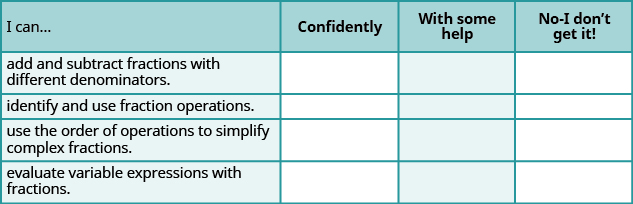After looking at the checklist, do you think you are well-prepared for the next chapter? Why or why not?

Aziza is solving this equation-2(1+x)=4x+10
No. 3^32 -1 has exactly two divisors greater than 75 and less than 85 what is their product?
x^2+7x-19=0 has Two solutions A and B give your answer to 3 decimal places
3. When Jenna spent 10 minutes on the elliptical trainer and then did circuit training for20 minutes, her fitness app says she burned 278 calories. When she spent 20 minutes onthe elliptical trainer and 30 minutes circuit training she burned 473 calories. How manycalories does she burn for each minute on the elliptical trainer? How many calories doesshe burn for each minute of circuit training?
.473
Angelita
?
Angelita
John left his house in Irvine at 8:35 am to drive to a meeting in Los Angeles, 45 miles away. He arrived at the meeting at 9:50. At 3:30 pm, he left the meeting and drove home. He arrived home at 5:18.
p-2/3=5/6 how do I solve it with explanation pls
P=3/2
Vanarith
1/2p2-2/3p=5p/6
James
Cindy
4.5
Ruth
is y=7/5 a solution of 5y+3=10y-4
yes
James
Cindy
Lucinda has a pocketful of dimes and quarters with a value of $6.20. The number of dimes is 18 more than 3 times the number of quarters. How many dimes and how many quarters does Lucinda have? Rhonda Reply Find an equation for the line that passes through the point P ( 0 , − 4 ) and has a slope 8/9 . Gabriel Reply is that a negative 4 or positive 4? Felix y = mx + b Felix if negative -4, then -4=8/9(0) + b Felix -4=b Felix if positive 4, then 4=b Felix then plug in y=8/9x - 4 or y=8/9x+4 Felix Macario is making 12 pounds of nut mixture with macadamia nuts and almonds. macadamia nuts cost$9 per pound and almonds cost $5.25 per pound. how many pounds of macadamia nuts and how many pounds of almonds should macario use for the mixture to cost$6.50 per pound to make?
Nga and Lauren bought a chest at a flea market for $50. They re-finished it and then added a 350 % mark - up Makaila Reply$1750
Cindy
the sum of two Numbers is 19 and their difference is 15
2, 17
Jose
interesting
saw
4,2
Cindy
Felecia left her home to visit her daughter, driving 45mph. Her husband waited for the dog sitter to arrive and left home 20 minutes, or 13 hour later. He drove 55mph to catch up to Felecia. How long before he reaches her?
hola saben como aser un valor de la expresión
NAILEA
integer greater than 2 and less than 12
2 < x < 12
Felix
I'm guessing you are doing inequalities...
Felix
Actually, translating words into algebraic expressions / equations...
Felix
hi
Darianna
hello
Mister
Eric here
Eric
6
Cindy

#### Get Jobilize Job Search Mobile App in your pocket Now!By Jonathan LongBy Steve GibbsBy John GabrieliBy OpenStaxBy Vanessa SoledadBy Cameron CaseyBy David GeltnerBy Brooke DelaneyBy Richley CrapoBy Marriyam Rana